Trapezoid MO-5-Z8

ABCD is a trapezoid that lime segment CE divided into a triangle and parallelogram as shown. Point F is the midpoint of CE, DF line passes through the center of the segment BE and the area of the triangle CDE is 3 cm2.
Determine the area of the trapezoid ABCD.

Result

S =  12 cm2

Solution:Leave us a comment of example and its solution (i.e. if it is still somewhat unclear...):

Showing 1 comment:Math studentNext similar examples:

1. 4-gonIt is true that a 4-gon whose two sides are parallel and the other two has equal length, is a parallelogram?
2. KLMN trapezoidThe KLMN trapezoid has bases KL 40cm and MN 16cm. On the KL base is point P. The segment NP divides the trapezoid into units with the same area. What is the distance of point P from point K?
3. Trapezoid MO-5-Z8Trapezoid KLMN has bases 12 and 4 cm long. The area of triangle KMN is 9 cm2. What is the area of the trapezoid KLMN?
4. Parcelparcel has a rectangular shape of a trapezoid with bases 12 m and 10 m and a height 8 m. On parcel was built object with a footprint an isosceles triangle shape with side 4 m and height three-quarters of a meter. What is the area of unbuild parcel?
5. Roof tilesThe roof has a trapezoidal shape with bases of 15 m and 10 m, height of roof is 4 meters. How many tiles will need if on 1 m2 should be used 8 tiles?
6. Parallelogram ABCDThe area of parallelogram ABCD is 440 cm2. Points M and N are the midpoints of the sides AB and BC. What is the area of a quadrilateral MBND?
7. Tunnels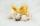Mice had built an underground house consisting of chambers and tunnels: • each tunnel leading from the chamber to the chamber (none is blind) • from each chamber lead just three tunnels into three distinct chambers, • from each chamber mice can get to any
8. Center traverseIt is true that the middle traverse bisects the triangle?
9. Katy MO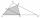Kate draw triangle ABC. Middle of AB have mark as X and the center of the side AC as Y. On the side BC wants to find the point Z such that the content area of a 4gon AXZY was greatest. What part of the triangle ABC can maximally occupy 4-gon AXZY?
10. Pyramid Z8–I–6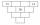Each brick of pyramid contains one number. Whenever possible, the number in each brick is lowest common multiple of two numbers of bricks lying directly above it. That number may be in the lowest brick? Determine all possibilities.
11. Skiing meeting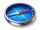On the skiing meeting came four friends from 4 world directions and led the next interview. Charles: "I did not come from the north or from the south." Mojmir "But I came from the south." Joseph: "I came from the north." Zdeno: "I come from the south." W
12. DiamondRhombus has side 17 cm and and one of diagonal 22 cm long. Calculate its area.
13. Diagonal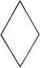Can be a diagonal of diamond twice longer than it side?
14. Parallelogram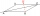Rhomboid (parallelogram) has a longer side of 50 cm long. The size of its one height is four times the size of its second height. Calculate the length of the shorter side of this rhomboid in the centimeters.
15. DiamondDetermine the side of diamond if its content is S = 353 cm2 and one diagonal u2 = 45 cm.
16. Circular lawnAround a circular lawn area is 2 m wide sidewalk. The outer edge of the sidewalk is curb whose width is 2 m. Curbstone and the inner side of the sidewalk together form a concentric circles. Calculate the area of the circular lawn and the result round to 1
17. Walnutsx walnuts were in the mission. Dano took 1/4 of nuts Michael took 1/8 from the rest and John took 34 nuts. It stayed here 29 nuts. Determine the original number of nuts.